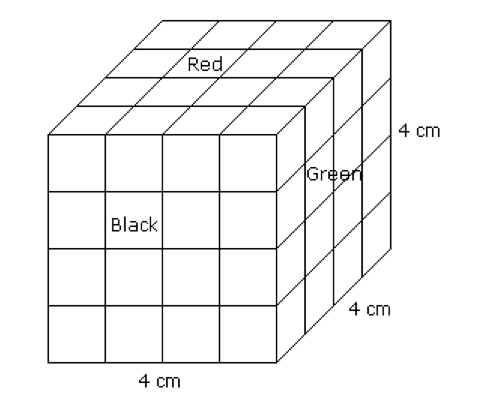## Cube & CuboidsIntroduction: In a cube or a cuboid there are six faces in each. In a cube length, breadth and height are same while in cuboid these are different. In a cube the number of unit cubes = (side)3. In cuboid the number of unit cube = (l x b x h). Example: A cube of …